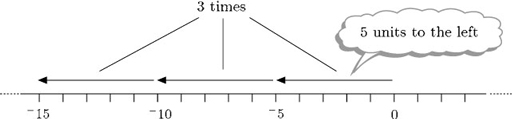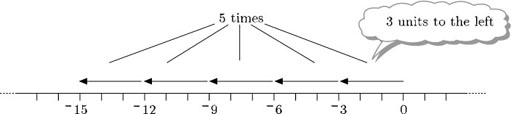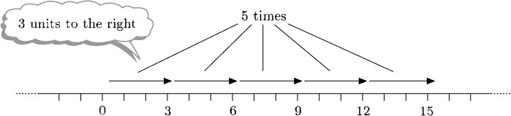Numbers, units and arithmetic

This free course is available to start right now. Review the full course description and key learning outcomes and create an account and enrol if you want a free statement of participation.

Free course

# 3.19 Multiplication with negative numbers

Now that you have rules for addition and subtraction of negative numbers, think about multiplication and division.

## Example 27

Describe each of the following in terms of the number line and the value of Thomas's piggy bank:

• (a) the multiplication of 3 times 5 (that is, 3 × 5);

• (b) the multiplication of 3 times 5 (that is, 3 × 5).

• (a) Three times 5 means adding three lots of 5, or do the move ‘5 units to the right’ three times.

• Thomas's piggy bank has three £5 notes in it. The value of the piggy bank in pounds is 3 × 5 = 15.

• (b) 3 times 5 means adding three lots of 5, or do 3 times ‘move 5 units to the left’.• Thomas's piggy bank has 3 IOUs each for £5 in it. The value of the piggy bank in pounds is 3 × 5 = 15.

So multiplying a negative number by a positive number gives a negative answer. But what does it mean to multiply by a negative number – how can you add something a negative number of times?

Think of multiplication by a positive number as repeated addition, and of multiplication by a negative number as repeated subtraction. If Thomas’ mother says she’ll take away three of his £5 IOUs, it is equivalent to giving him three £5 notes. So 3 × 5 = 3 × 5 = 15. He is £15 better off.

## Example 28

Evaluate each of the following in terms of the number line and the value of Thomas’s piggy bank.

• (a) 5 × 3

• (b) 5 × 3

• (a) Think of 5 times 3 as meaning ‘5 lots of 3’. So 5 × 3 means: do 5 times ‘move 3 units to the left’.• So 5 × 3 = 15.

• In terms of the piggy bank Thomas has five £3 IOUs in it. The value in pounds is 5 × 3 = 15, which is equivalent to one £15 IOU.

• (b) Thinking in terms of repeated subtraction, do 5 times ‘subtract 3’. Subtracting 3 means adding 3, or moving 3 units to the right. So do 5 times ‘move 3 units to the right’.

Subtracting 3 is the same as adding 3.• So 5 × 3 = 5 × 3 = 15.

• Thomas's mother takes away five £3 IOUs. This is equivalent to giving him £15.

• The calculation in pounds is 5 × 3 = 5 × 3 = 15.

MU120_4M1